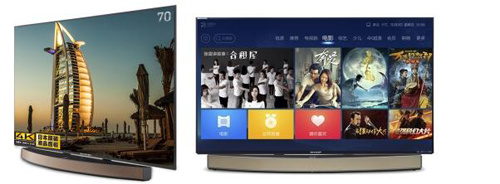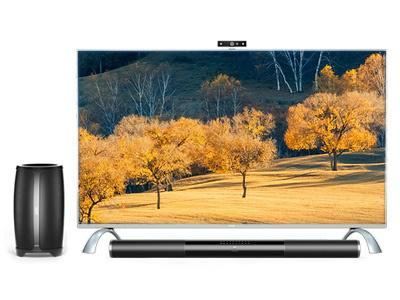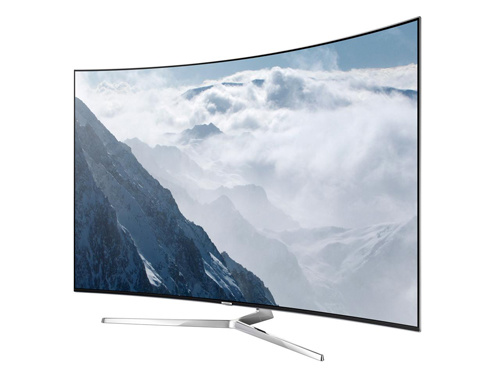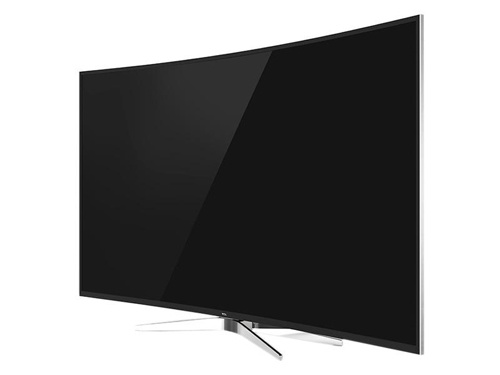|

# 巨屏电视3米观看距离可享受大屏时代最佳智能电视选购

根据以往的观点，电视屏幕尺寸越大，使用者距离屏幕的距离应该越远。这并不是一个错误的观点，这样划分屏幕与观看距离的关系，结症所在是保证屏幕分辨率能够足够清晰的呈现在用户面前。

随着液晶面板屏幕分辩的不断提升，观看距离已经不再受观看距离制约，4K级别的巨屏电视，3米之内的观看距离都能有非常出色的显示效果。所以消费者不用纠结自家客厅不够宽敞，趁着双十一期间，我们盘点一些巨屏电视来装饰自家客厅吧。毕竟，双十一是液晶市场最后的降价期，全球液晶面板整体缺货，成品电视价格水涨船高已经愈发明显，所以趁着这波降价潮，赶紧给自己的客厅升级吧。

夏普LCD-70XT85A与富士康联姻之后，夏普近期一口气推出了四款新品电视，其中70吋产品是旗舰型号，该机在夏普天猫旗舰店原价16999元，双十一旗舰通过官方活动，可以13999元的价格购买，同时还可获赠60吋夏普智能电视一台，相当于13999元购买70吋电视+60吋电视，这是目前双十一期间电视领域优惠力度最猛的一款。夏普LCD-70XT85A采用分离式设计，处理核心与音响系统与屏幕分开。分离式电视最大的优势在于，用户可以根据自身的喜好，更新升级硬件、存储、音响系统等，不仅大大提升了电视的可玩性，同时也让自家客厅电视一直处于最顶级配置。得益于分离式设计，夏普LCD-70XT85A机身厚度最薄处仅9.7mm，不含底座机身重量约为27公斤，这样的机身三围在立式、壁挂式两种形态上，都能有着不错的发挥。

在液晶背板方面，夏普LCD-70XT85A配置全球领先的十代线面板，其色彩饱和度、亮度都会给消费者带来极佳的观看感受。该机屏幕尺寸达到70吋，屏幕分辨率为Ultra HD级别的3840 X 2160像素，是一款4K级别产品，屏幕分辨率达到1080P产品的四倍。通过夏普独有的色彩智能调节功能，显示效果细腻自然，同时有效保护眼球健康。搭配底座上的独立印象单元，70吋4K分辨率的夏普LCD-70XT85A可以为客厅带来家庭影院版的视听效果。

夏普LCD-70XT85A是一款纯粹的互联网智能电视，该机不仅支持WLAN无线数据连接和LAN端口有线连接，来自阿里的Yun OS for TV系统，不仅为该机提供了海量的视频源，同时支持应用程序扩展。用户可以在电视上实现网络购物、玩更多的电视游戏等等。通过夏普天猫旗舰店了解到，夏普LCD-70XT85A赠送730天优酷土豆VIP会员，热剧、独播剧、热门综艺随时观看。作为一款4K电视，除外接存储设备播放4K片源外，Yun OS for TV为消费者准备了4K专区，4K片源一网打尽。

综合来看，夏普LCD-70XT85A在双十一期间优惠力度颇为丰厚，购买70吋电视还可获赠60吋4K电视，一台放在客厅，一台放在卧室，加上夏普品牌和堺十代线的强大支持，夏普LCD-70XT85A或许会成为双十一爆款产品。

夏普LCD-70XT85A

售价 13999元（获赠60吋夏普电视）

购买渠道 夏普天猫旗舰店

乐视超级电视 超4 Max70-3D乐视超级电视依托乐视影业出色的视频资源，发布之后受到了不少消费者的青睐。最新发布的超4 Max70-3D顾名思义，这是一款70英寸4K分辨率3D电视，超4 Max70-3D液晶面板来自夏普十代线，机身采用分体式设计，双十一期间以14999元的价格进行抢购，而分体底座需要单独购买。

外观方面，超4延续乐视电视一贯的科幻风格，流线型底座给人第一印象很好。70英寸屏幕非常轻薄，方便立式或者悬挂。独立底座承载音箱、核心处理等工作。整体看乐视超4是一款非常适合在家庭中使用的产品。

硬件方面，超4采用夏普十代线面板，分辨率达到了4K级别，支持3D显示功能，核心硬件搭载高通公司的8094处理器，性能表现非常理想。搭配Android系统，用户在电视上可以实现更多的应用程序扩展。内容方面，乐视超4享受乐视网、乐视影业、乐视体育提供的海量内容源，无论是追剧、综艺还是享受体育栏目，都会有不错的收获。

作为时下最热门的智能电视，超4 70-3D整体配置非常突出，双十一期间京东可以14999元价格抢购，但机身底座需要另行购买。

乐视超级电视 超4 70-3D

售价14999元（底座另行购买）

购买渠道：京东

三星UA65KS9800三星作为全球消费电子巨头，在电视领域始终勤奋耕耘。从最早的智能电视、UHD超高清电视、曲面UHD电视到全新量子点电视都有涉猎。2016年，三星带来全新第二代SUHD量子点电视UA65KS9800，目前该机在国美在线售价26499元，想要感受曲面电视的朋友不妨考虑一下。

KS9800第二代曲面量子点电视做工十分考究。这款SUHD电视采用曲面设计，不仅外观造型别致，还可增强观看的纵深感与立体感，整体做工相当考究，KS9800作为旗舰机型，产品整体的科技感十足。

KS9800系列电视搭载曲率为4200的无镉10bit第二代量子点显示屏，同时配合三星的深黑减反、自动景深技术，大大减少了屏幕炫光、增加画面层次感，保证从不同角度观看都能够获得近似一致的观影体验。接口方面，KS9800提供了4个HDMI、3个USB、1个分量输入、1个AV输入、1个LAN网线插口、1个数字音频光纤输出以及1个射频接口，足以应对日常使用。

三星UA65KS9800

售价：26499元

购买渠道：国美在线

TCL L65C2-CUDGTCL 65英寸C2曲面电视拥有ViewMax黄金视域设计，视觉效果非常理想。外观设计上通过突破性的全新设计，将厚度减少至12mm，同时在设计上结合轻奢格调与微观美学，幻月浮空底座与轻薄机身。在硬件配置方面，64位智能机芯，14核强劲配置，让你尽情享受智能电视带来的乐趣。

L65C2电视融入全生态HDR技术， 显示亮度答复提高，通过对亮度的提升，能够极大的提高对比度，有效提升画质效果。而4000R黄金曲率屏幕的创造性应用，不但提升屏幕视野范围，形成沉浸式的视听体验。

这款电视专为运动而生，搭载了第二代C运动，配备了羽毛球、网球、保龄球、瑜伽、慢跑等体育项目。作为TCL全新一代TV+产品，L65C2搭载了腾讯视频覆盖海量资源，让用户看到院线同期的热映电影。更有C运动、歌友会、微信互联等各种应用程序，让用户从此畅享全新的家庭娱乐体验。

TCL L65C2-CUDG

售价：16999元

购买渠道：京东

`声明：本文由入驻焦点开放平台的作者撰写，除焦点官方账号外，观点仅代表作者本人，不代表焦点立场错误信息举报电话： 400-099-0099，邮箱：jubao@vip.sohu.com，或点此进行意见反馈，或点此进行举报投诉。`A B C D E F G H J K L M N P Q R S T W X Y Z
A - B - C - D - E
• A
• 鞍山
• 安庆
• 安阳
• 安顺
• 安康
• 澳门
• B
• 北京
• 保定
• 包头
• 巴彦淖尔
• 本溪
• 蚌埠
• 亳州
• 滨州
• 北海
• 百色
• 巴中
• 毕节
• 保山
• 宝鸡
• 白银
• 巴州
• C
• 承德
• 沧州
• 长治
• 赤峰
• 朝阳
• 长春
• 常州
• 滁州
• 池州
• 长沙
• 常德
• 郴州
• 潮州
• 崇左
• 重庆
• 成都
• 楚雄
• 昌都
• 慈溪
• 常熟
• D
• 大同
• 大连
• 丹东
• 大庆
• 东营
• 德州
• 东莞
• 德阳
• 达州
• 大理
• 德宏
• 定西
• 儋州
• 东平
• E
• 鄂尔多斯
• 鄂州
• 恩施
F - G - H - I - J
• F
• 抚顺
• 阜新
• 阜阳
• 福州
• 抚州
• 佛山
• 防城港
• G
• 赣州
• 广州
• 桂林
• 贵港
• 广元
• 广安
• 贵阳
• 固原
• H
• 邯郸
• 衡水
• 呼和浩特
• 呼伦贝尔
• 葫芦岛
• 哈尔滨
• 黑河
• 淮安
• 杭州
• 湖州
• 合肥
• 淮南
• 淮北
• 黄山
• 菏泽
• 鹤壁
• 黄石
• 黄冈
• 衡阳
• 怀化
• 惠州
• 河源
• 贺州
• 河池
• 海口
• 红河
• 汉中
• 海东
• 怀来
• I
• J
• 晋中
• 锦州
• 吉林
• 鸡西
• 佳木斯
• 嘉兴
• 金华
• 景德镇
• 九江
• 吉安
• 济南
• 济宁
• 焦作
• 荆门
• 荆州
• 江门
• 揭阳
• 金昌
• 酒泉
• 嘉峪关
K - L - M - N - P
• K
• 开封
• 昆明
• 昆山
• L
• 廊坊
• 临汾
• 辽阳
• 连云港
• 丽水
• 六安
• 龙岩
• 莱芜
• 临沂
• 聊城
• 洛阳
• 漯河
• 娄底
• 柳州
• 来宾
• 泸州
• 乐山
• 六盘水
• 丽江
• 临沧
• 拉萨
• 林芝
• 兰州
• 陇南
• M
• 牡丹江
• 马鞍山
• 茂名
• 梅州
• 绵阳
• 眉山
• N
• 南京
• 南通
• 宁波
• 南平
• 宁德
• 南昌
• 南阳
• 南宁
• 内江
• 南充
• P
• 盘锦
• 莆田
• 平顶山
• 濮阳
• 攀枝花
• 普洱
• 平凉
Q - R - S - T - W
• Q
• 秦皇岛
• 齐齐哈尔
• 衢州
• 泉州
• 青岛
• 清远
• 钦州
• 黔南
• 曲靖
• 庆阳
• R
• 日照
• 日喀则
• S
• 石家庄
• 沈阳
• 双鸭山
• 绥化
• 上海
• 苏州
• 宿迁
• 绍兴
• 宿州
• 三明
• 上饶
• 三门峡
• 商丘
• 十堰
• 随州
• 邵阳
• 韶关
• 深圳
• 汕头
• 汕尾
• 三亚
• 三沙
• 遂宁
• 山南
• 商洛
• 石嘴山
• T
• 天津
• 唐山
• 太原
• 通辽
• 铁岭
• 泰州
• 台州
• 铜陵
• 泰安
• 铜仁
• 铜川
• 天水
• 天门
• W
• 乌海
• 乌兰察布
• 无锡
• 温州
• 芜湖
• 潍坊
• 威海
• 武汉
• 梧州
• 渭南
• 武威
• 吴忠
• 乌鲁木齐
X - Y - Z
• X
• 邢台
• 徐州
• 宣城
• 厦门
• 新乡
• 许昌
• 信阳
• 襄阳
• 孝感
• 咸宁
• 湘潭
• 湘西
• 西双版纳
• 西安
• 咸阳
• 西宁
• 仙桃
• 西昌
• Y
• 运城
• 营口
• 盐城
• 扬州
• 鹰潭
• 宜春
• 烟台
• 宜昌
• 岳阳
• 益阳
• 永州
• 阳江
• 云浮
• 玉林
• 宜宾
• 雅安
• 玉溪
• 延安
• 榆林
• 银川
• Z
• 张家口
• 镇江
• 舟山
• 漳州
• 淄博
• 枣庄
• 郑州
• 周口
• 驻马店
• 株洲
• 张家界
• 珠海
• 湛江
• 肇庆
• 中山
• 自贡
• 资阳
• 遵义
• 昭通
• 张掖
• 中卫

1室1厅1厨1卫1阳台

1
2
3
4
5

0
1
2

1

1

0
1
2
3报名成功，资料已提交审核A B C D E F G H J K L M N P Q R S T W X Y Z
A - B - C - D - E
• A
• 鞍山
• 安庆
• 安阳
• 安顺
• 安康
• 澳门
• B
• 北京
• 保定
• 包头
• 巴彦淖尔
• 本溪
• 蚌埠
• 亳州
• 滨州
• 北海
• 百色
• 巴中
• 毕节
• 保山
• 宝鸡
• 白银
• 巴州
• C
• 承德
• 沧州
• 长治
• 赤峰
• 朝阳
• 长春
• 常州
• 滁州
• 池州
• 长沙
• 常德
• 郴州
• 潮州
• 崇左
• 重庆
• 成都
• 楚雄
• 昌都
• 慈溪
• 常熟
• D
• 大同
• 大连
• 丹东
• 大庆
• 东营
• 德州
• 东莞
• 德阳
• 达州
• 大理
• 德宏
• 定西
• 儋州
• 东平
• E
• 鄂尔多斯
• 鄂州
• 恩施
F - G - H - I - J
• F
• 抚顺
• 阜新
• 阜阳
• 福州
• 抚州
• 佛山
• 防城港
• G
• 赣州
• 广州
• 桂林
• 贵港
• 广元
• 广安
• 贵阳
• 固原
• H
• 邯郸
• 衡水
• 呼和浩特
• 呼伦贝尔
• 葫芦岛
• 哈尔滨
• 黑河
• 淮安
• 杭州
• 湖州
• 合肥
• 淮南
• 淮北
• 黄山
• 菏泽
• 鹤壁
• 黄石
• 黄冈
• 衡阳
• 怀化
• 惠州
• 河源
• 贺州
• 河池
• 海口
• 红河
• 汉中
• 海东
• 怀来
• I
• J
• 晋中
• 锦州
• 吉林
• 鸡西
• 佳木斯
• 嘉兴
• 金华
• 景德镇
• 九江
• 吉安
• 济南
• 济宁
• 焦作
• 荆门
• 荆州
• 江门
• 揭阳
• 金昌
• 酒泉
• 嘉峪关
K - L - M - N - P
• K
• 开封
• 昆明
• 昆山
• L
• 廊坊
• 临汾
• 辽阳
• 连云港
• 丽水
• 六安
• 龙岩
• 莱芜
• 临沂
• 聊城
• 洛阳
• 漯河
• 娄底
• 柳州
• 来宾
• 泸州
• 乐山
• 六盘水
• 丽江
• 临沧
• 拉萨
• 林芝
• 兰州
• 陇南
• M
• 牡丹江
• 马鞍山
• 茂名
• 梅州
• 绵阳
• 眉山
• N
• 南京
• 南通
• 宁波
• 南平
• 宁德
• 南昌
• 南阳
• 南宁
• 内江
• 南充
• P
• 盘锦
• 莆田
• 平顶山
• 濮阳
• 攀枝花
• 普洱
• 平凉
Q - R - S - T - W
• Q
• 秦皇岛
• 齐齐哈尔
• 衢州
• 泉州
• 青岛
• 清远
• 钦州
• 黔南
• 曲靖
• 庆阳
• R
• 日照
• 日喀则
• S
• 石家庄
• 沈阳
• 双鸭山
• 绥化
• 上海
• 苏州
• 宿迁
• 绍兴
• 宿州
• 三明
• 上饶
• 三门峡
• 商丘
• 十堰
• 随州
• 邵阳
• 韶关
• 深圳
• 汕头
• 汕尾
• 三亚
• 三沙
• 遂宁
• 山南
• 商洛
• 石嘴山
• T
• 天津
• 唐山
• 太原
• 通辽
• 铁岭
• 泰州
• 台州
• 铜陵
• 泰安
• 铜仁
• 铜川
• 天水
• 天门
• W
• 乌海
• 乌兰察布
• 无锡
• 温州
• 芜湖
• 潍坊
• 威海
• 武汉
• 梧州
• 渭南
• 武威
• 吴忠
• 乌鲁木齐
X - Y - Z
• X
• 邢台
• 徐州
• 宣城
• 厦门
• 新乡
• 许昌
• 信阳
• 襄阳
• 孝感
• 咸宁
• 湘潭
• 湘西
• 西双版纳
• 西安
• 咸阳
• 西宁
• 仙桃
• 西昌
• Y
• 运城
• 营口
• 盐城
• 扬州
• 鹰潭
• 宜春
• 烟台
• 宜昌
• 岳阳
• 益阳
• 永州
• 阳江
• 云浮
• 玉林
• 宜宾
• 雅安
• 玉溪
• 延安
• 榆林
• 银川
• Z
• 张家口
• 镇江
• 舟山
• 漳州
• 淄博
• 枣庄
• 郑州
• 周口
• 驻马店
• 株洲
• 张家界
• 珠海
• 湛江
• 肇庆
• 中山
• 自贡
• 资阳
• 遵义
• 昭通
• 张掖
• 中卫• 手机• 分享
• 设计
免费设计
• 计算器
装修计算器
• 入驻
合作入驻
• 联系
联系我们
• 置顶
返回顶部